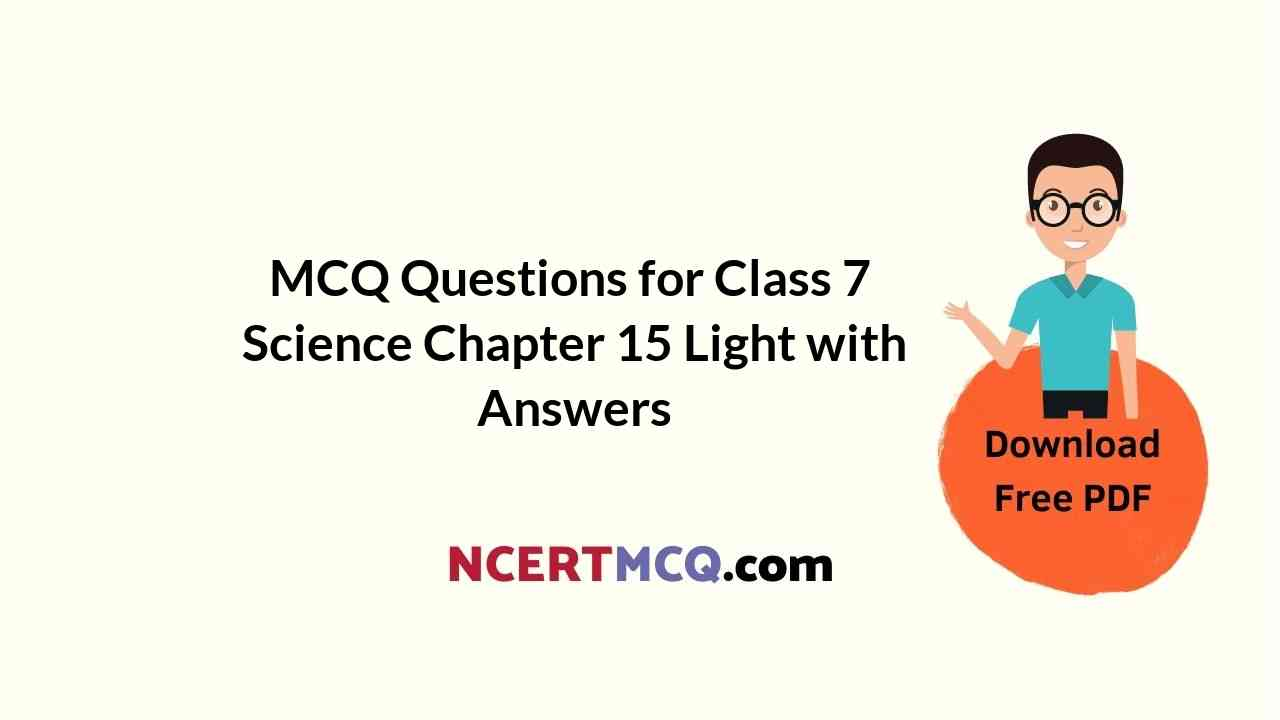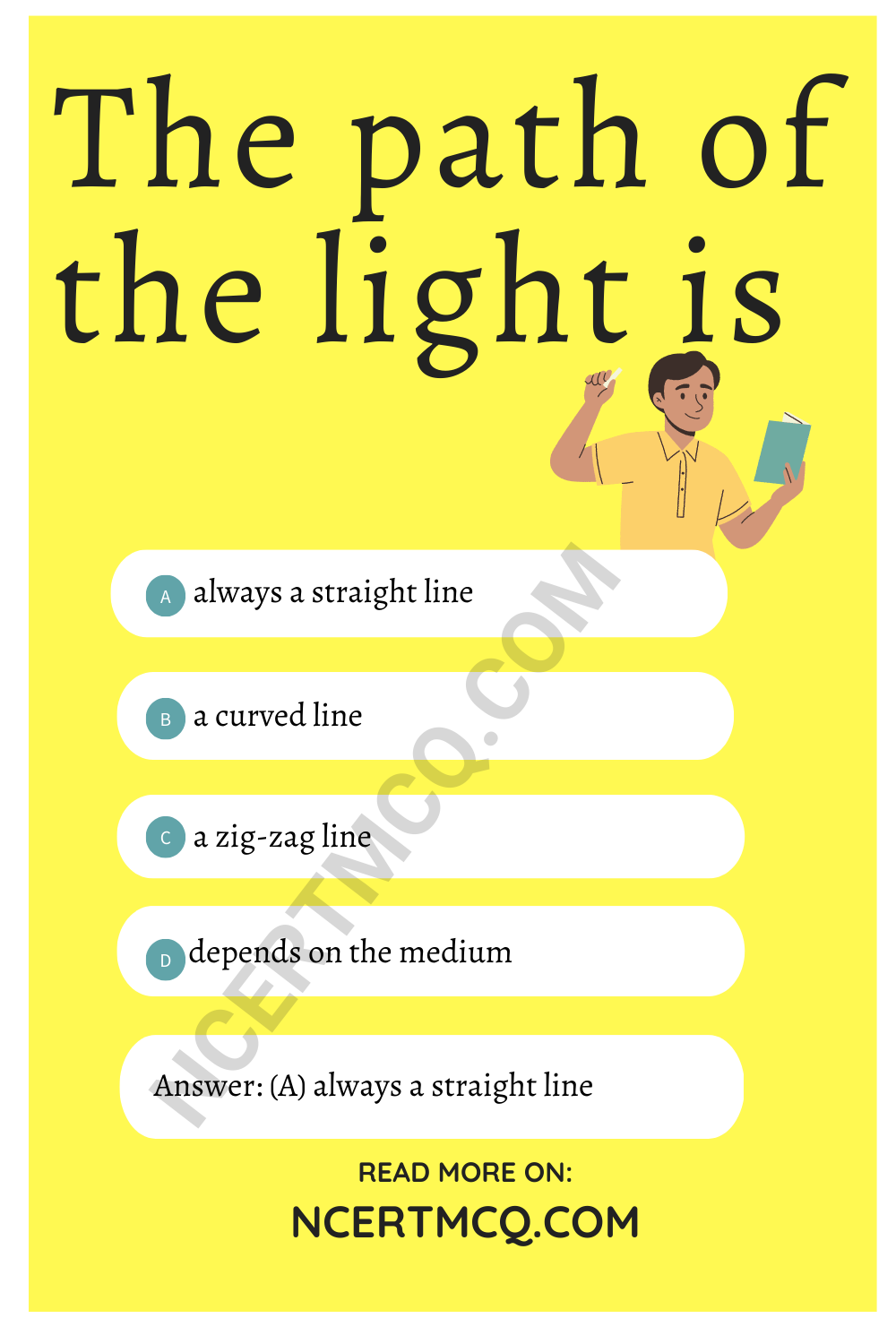Check the below Online Education NCERT MCQ Questions for Class 7 Science Chapter 15 Light with Answers Pdf free download. MCQ Questions for Class 7 Science with Answers were prepared based on the latest exam pattern. We have Provided Light Class 7 Science MCQs Questions with Answers to help students understand the concept very well. https://ncertmcq.com/mcq-questions-for-class-7-science-with-answers/

You can refer to NCERT Solutions for Class 7 Science Chapter 15 Light to revise the concepts in the syllabus effectively and improve your chances of securing high marks in your board exams.

## Light Class 7 MCQs Questions with Answers

Light Class 7 MCQ Chapter 15 Question 1.
The path of the light is
(a) always a straight line
(b) a curved line
(c) a zig-zag line
(d) depends on the medium

Answer: (a) always a straight lineLight MCQ Class 7 Chapter 15 Question 2.
Which one shows lateral inversion?
(a) Plane mirror
(b) Convex mirror
(c) Concave mirror
(d) All of these

Light Class 7 MCQ With Answers Chapter 15 Question 3.
Image formed by a plane mirror is
(a) virtual and erect
(b) real and erect
(c) virtual and inverted
(d) real and inverted

Class 7 Science Chapter 15 MCQ Question 4.
Boojho and Paheli were given one mirror each by their teacher. Boojho found his image to be erect and of the same size whereas Paheli found her image erect and smaller in size. This means that the mirrors of Boojho and Paheli are, respectively
(a) plane mirror and concave mirror
(b) concave mirror and convex mirror
(c) plane mirror and convex mirror
(d) convex mirror and plane mirror

Answer: (c) plane mirror and convex mirror

Class 7 Light MCQ Chapter 15 Question 5.
Which of the following can be used to form a real image?
(a) Concave mirror only
(b) Plane mirror only
(c) Convex mirror only
(d) Both concave and convex mirrors

MCQ On Light Class 7 Chapter 15 Question 6.
If an object is placed at a distance of 0.5 m in front of a plane mirror, the distance between the object and the image formed by the mirror will be
(a) 2 m
(b) 1 m
(c) 0.5 m
(d) 0.25 m

MCQ Of Light Class 7 Chapter 15 Question 7.
You are provided with a concave mirror, a convex mirror, a concave lens and a convex lens. To obtain an enlarged image of an object you can use either
(a) concave mirror or convex mirror
(b) concave mirror or convex lens
(c) concave mirror or concave lens
(d) concave lens or convex lens

Answer: (b) concave mirror or convex lens

MCQ On Light For Class 7 With Answers Chapter 15 Question 8.
An erect and enlarged image can be formed by
(a) only a convex mirror
(b) only a concave mirror
(c) only a plane mirror
(d) both convex and concave mirror

Answer: (b) only a concave mirror

Class 7 Science Ch 15 MCQ Question 9.
You are provided with a convex mirror, a concave mirror, a convex lens and a concave lens. You can get an inverted image from
(a) both concave lens and convex lens
(b) both concave mirror and convex mirror
(c) both convex mirror and convex lens
(d) both convex mirror and concave lens

Answer: (c) both convex mirror and convex lens

Light Chapter Class 7 MCQ Chapter 15 Question 10.
An image formed by a lens is erect. Such an image could be formed by a
(a) convex lens provided the image is smaller than object.
(b) concave lens provided the image is smaller than object.
(c) concave lens provided the image is larger than object.
(d) concave lens provided the image is of the same size.

Answer: (b) concave lens provided the image is smaller than object.Match the following:

 Column A Column B (i) Concave lens (a) Lateral inversion (ii) Always virtual image (b) Reflecting surface is bulged out (iii) Changing position of image (c) Concave mirror (iv) Wax paper (d) Seven colours (v) Image obtained on screen (e) Real image (vi) Convex mirror (f) Translucent (vii) Used by dentists (g) Thinner in the middle (viii) White light composed (h) Concave lens

 Column A Column B (i) Concave lens (g) Thinner in the middle (ii) Always virtual image (h) Concave lens (iii) Changing position of image (a) Lateral inversion (iv) Wax paper (f) Translucent (v) Image obtained on screen (e) Real image (vi) Convex mirror (b) Reflecting surface is bulged out (vii) Used by dentists (c) Concave mirror (viii) White light composed (d) Seven colours

Fill in the blanks:

1. Uneven surfaces show ………………….. reflection.

2. Incident ray, the reflected ray ………………….. and lie in the same …………………..

3. Laws of ………………….. is applicable in playing back shots in the carroms.

4. Changing of right side to left is called …………………..

5. The inner surface of a steel spoon acts as a ………………….. mirror.

6. The outer surface of a flat steel plate acts as a ………………….. mirror.

7. The outer shining surface of a round bottom steel bowl acts as a ………………….. mirror.

8. The inner surface of the reflector of a torch acts as a ………………….. mirror.

Choose the true and false statements from the following:

1. Concave mirror is converging in nature.

2. Convex mirror is diverging in nature.

3. Plane mirror forms virtual image.

4. Concave mirror has a virtual focus.

5. Spherical mirrors do not obey laws of reflection.

6. During lateral inversion, the image becomes inverted.